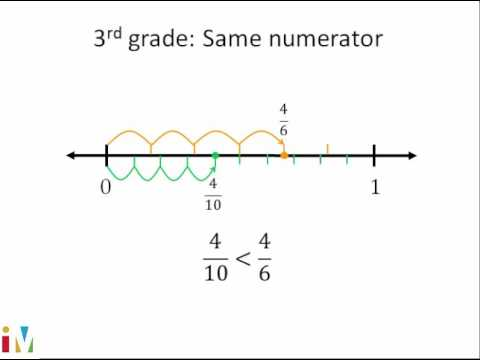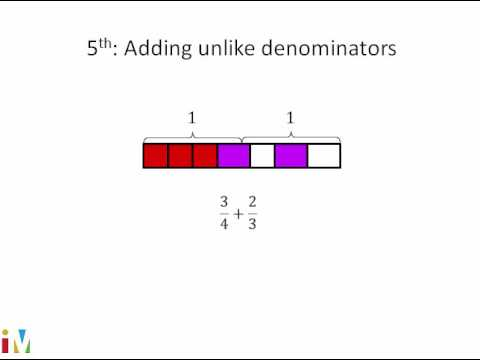Video

# Understanding fractions as division (Full video)

Description: Learn how a/b and a ÷ b are equivalent. (That is, the fraction bar and the division symbol mean the same thing.) And we learned many, many videos ago that this, of course, is going to be equal to 8. So let's start with one, two, three, four, five, six, seven, eight things. And we could say, well, let's try to divide that into four groups, four equal groups.

### Other videos you might be interested in### Comparing Fractions

#### Illustrative Mathematics